Info

Tip

1、路径：也就是已经做出的选择。

2、选择列表：也就是你当前可以做的选择。

3、结束条件：也就是到达决策树底层，无法再做选择的条件。

``````result = []
def backtrack(路径, 选择列表):
if 满足结束条件:
return

for 选择 in 选择列表:
做选择
backtrack(路径, 选择列表)
撤销选择
``````

### # 一、全排列问题

Info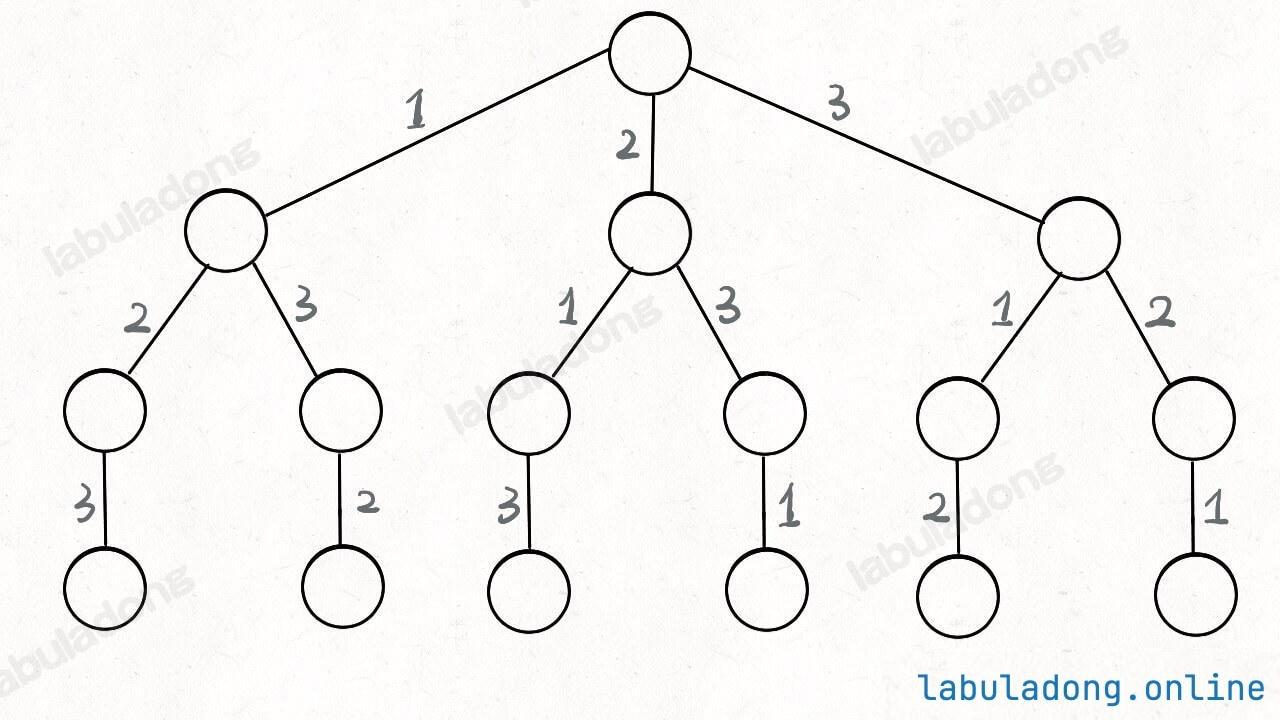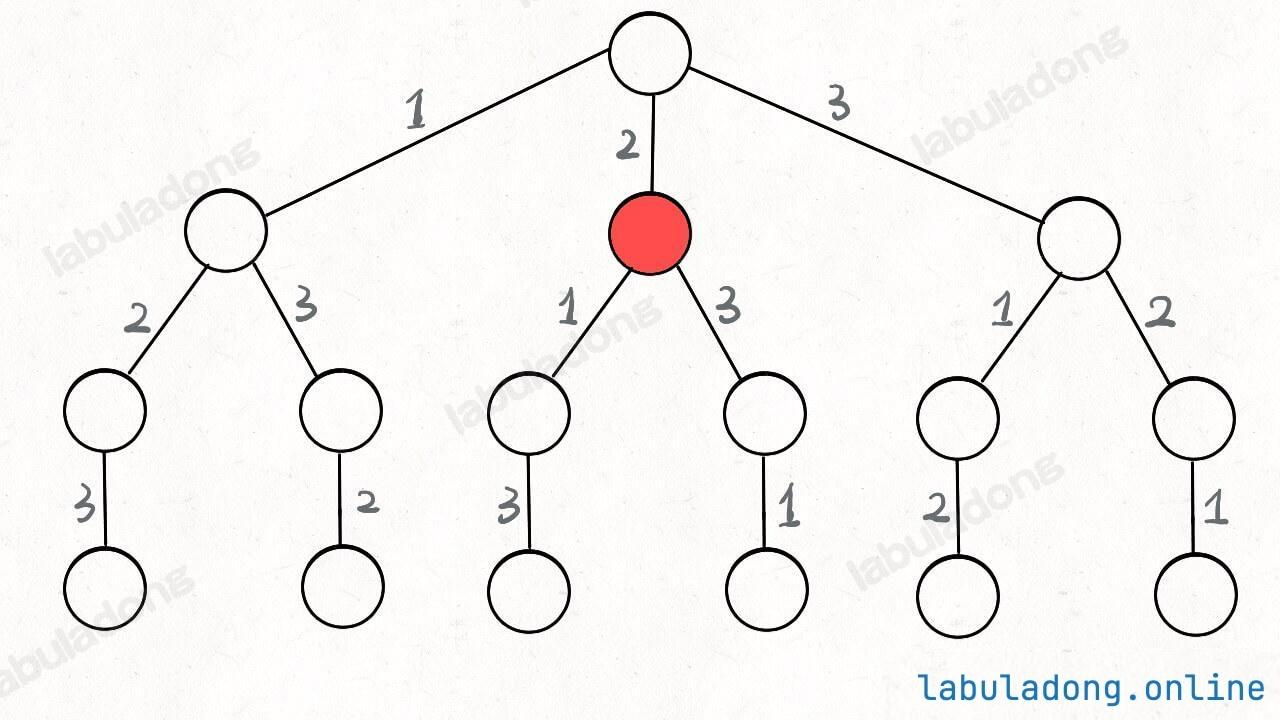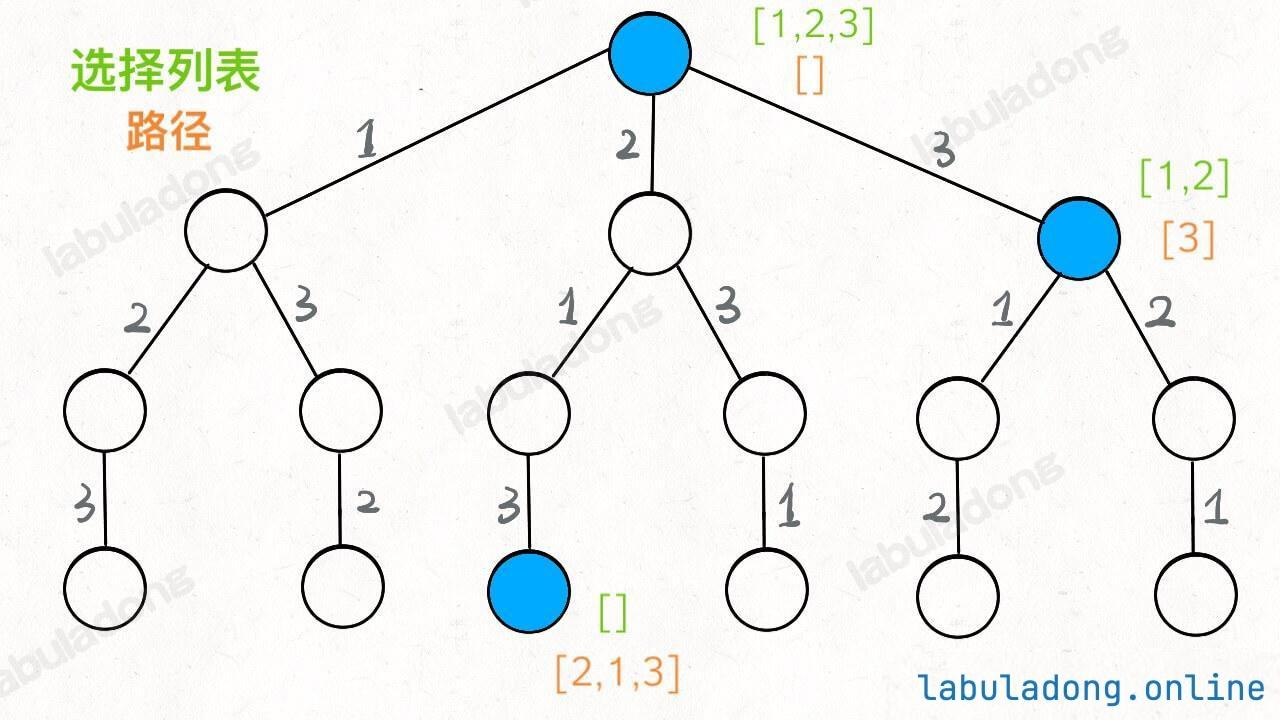``````void traverse(TreeNode root) {
for (TreeNode child : root.childern) {
// 前序位置需要的操作
traverse(child);
// 后序位置需要的操作
}
}
``````

Info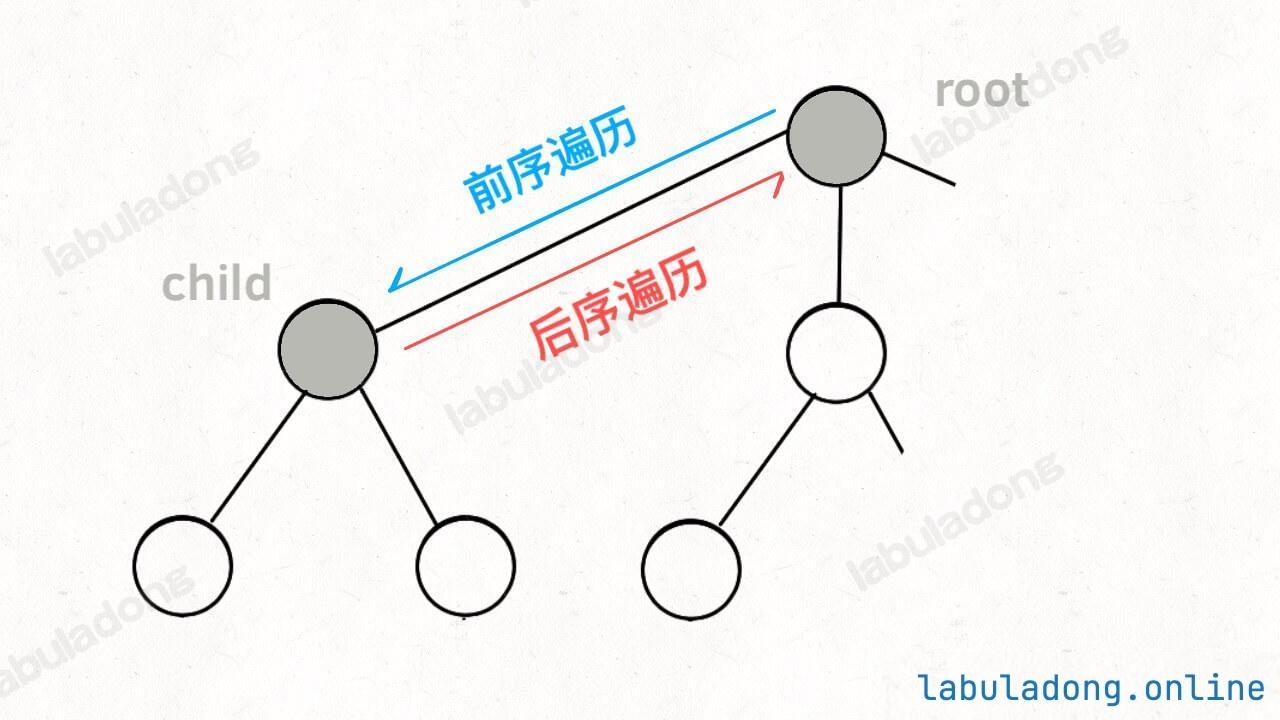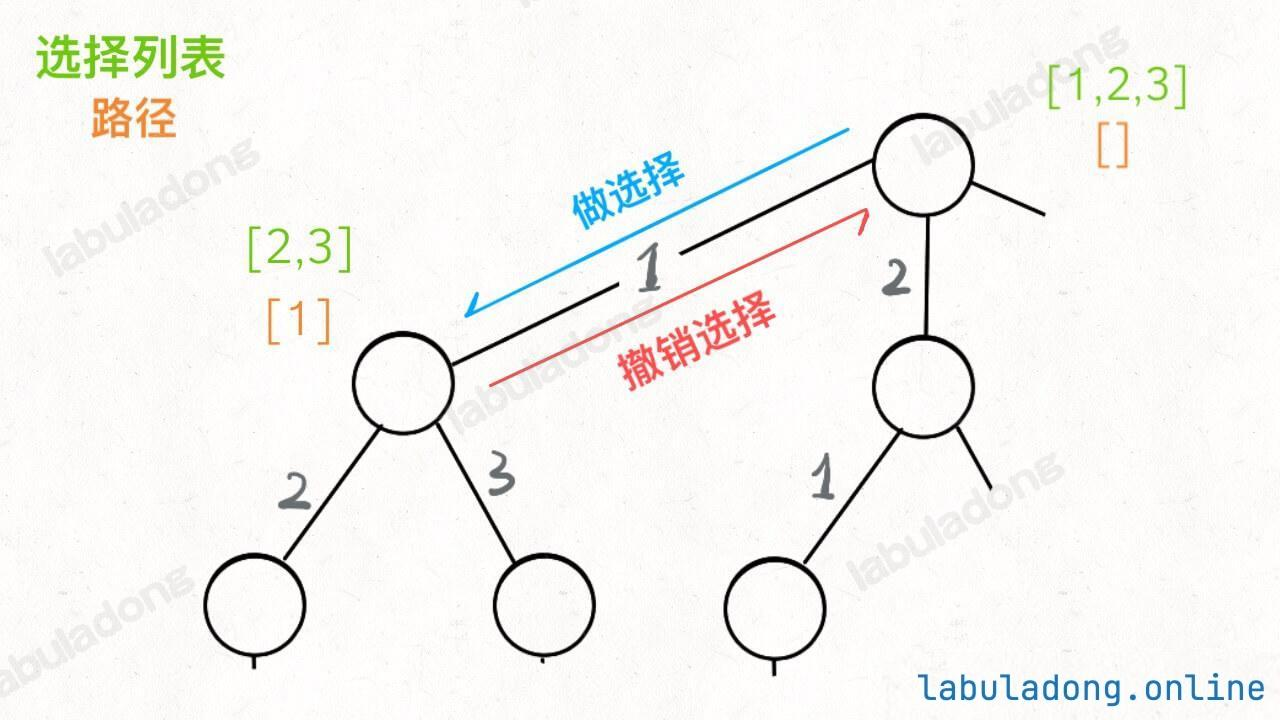``````for 选择 in 选择列表:
# 做选择
将该选择从选择列表移除
backtrack(路径, 选择列表)
# 撤销选择
路径.remove(选择)
将该选择再加入选择列表
``````

``````class Solution {

/* 主函数，输入一组不重复的数字，返回它们的全排列 */
List<List<Integer>> permute(int[] nums) {
// 记录「路径」
// 「路径」中的元素会被标记为 true，避免重复使用
boolean[] used = new boolean[nums.length];

backtrack(nums, track, used);
return res;
}

// 路径：记录在 track 中
// 选择列表：nums 中不存在于 track 的那些元素（used[i] 为 false）
// 结束条件：nums 中的元素全都在 track 中出现
void backtrack(int[] nums, LinkedList<Integer> track, boolean[] used) {
// 触发结束条件
if (track.size() == nums.length) {
return;
}

for (int i = 0; i < nums.length; i++) {
// 排除不合法的选择
if (used[i]) {/**<extend up -200><div class="img-content"><img src="/algo/images/backtracking/6.jpg" class="myimage"/></div> */
// nums[i] 已经在 track 中，跳过
continue;
}
// 做选择
used[i] = true;
// 进入下一层决策树
backtrack(nums, track, used);
// 取消选择
track.removeLast();
used[i] = false;
}
}
}
``````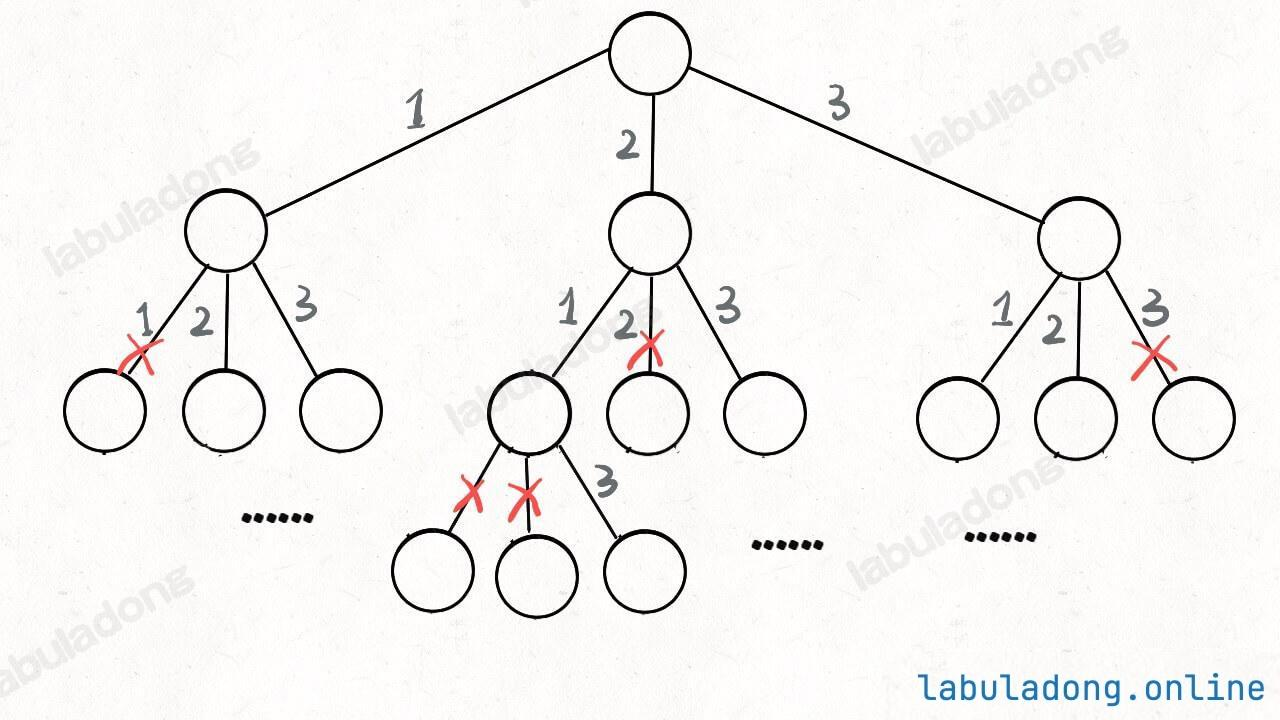### # 二、N 皇后问题

``````vector<vector<string>> solveNQueens(int n);
``````

Tip

``````[
[".Q..","...Q","Q...","..Q."],
["..Q.","Q...","...Q",".Q.."]
]
``````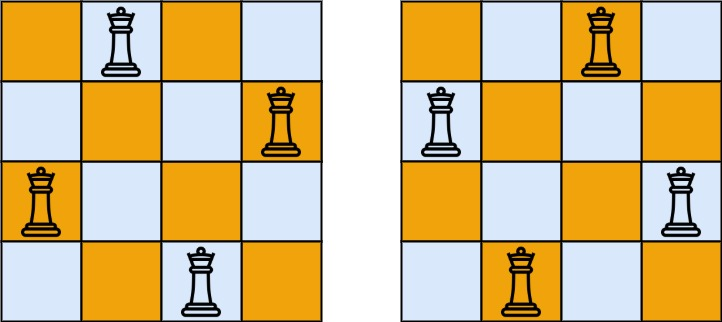``````class Solution {
public:
vector<vector<string>> res;

/* 输入棋盘边长 n，返回所有合法的放置 */
vector<vector<string>> solveNQueens(int n) {
// vector<string> 代表一个棋盘
// '.' 表示空，'Q' 表示皇后，初始化空棋盘
vector<string> board(n, string(n, '.'));
backtrack(board, 0);
return res;
}

// 路径：board 中小于 row 的那些行都已经成功放置了皇后
// 选择列表：第 row 行的所有列都是放置皇后的选择
// 结束条件：row 超过 board 的最后一行
void backtrack(vector<string>& board, int row) {
// 触发结束条件
if (row == board.size()) {
res.push_back(board);
return;
}

int n = board[row].size();
for (int col = 0; col < n; col++) {
// 排除不合法选择
if (!isValid(board, row, col)) {/**<extend up -200><div class="img-content"><img src="/algo/images/backtracking/7.jpg" class="myimage"/></div> */
continue;
}
// 做选择
board[row][col] = 'Q';
// 进入下一行决策
backtrack(board, row + 1);
// 撤销选择
board[row][col] = '.';
}
}
}
``````

``````/* 是否可以在 board[row][col] 放置皇后？ */
bool isValid(vector<string>& board, int row, int col) {
int n = board.size();
// 检查列是否有皇后互相冲突
for (int i = 0; i <= row; i++) {
if (board[i][col] == 'Q')
return false;
}
// 检查右上方是否有皇后互相冲突
for (int i = row - 1, j = col + 1;
i >= 0 && j < n; i--, j++) {
if (board[i][j] == 'Q')
return false;
}
// 检查左上方是否有皇后互相冲突
for (int i = row - 1, j = col - 1;
i >= 0 && j >= 0; i--, j--) {
if (board[i][j] == 'Q')
return false;
}
return true;
}
``````

Info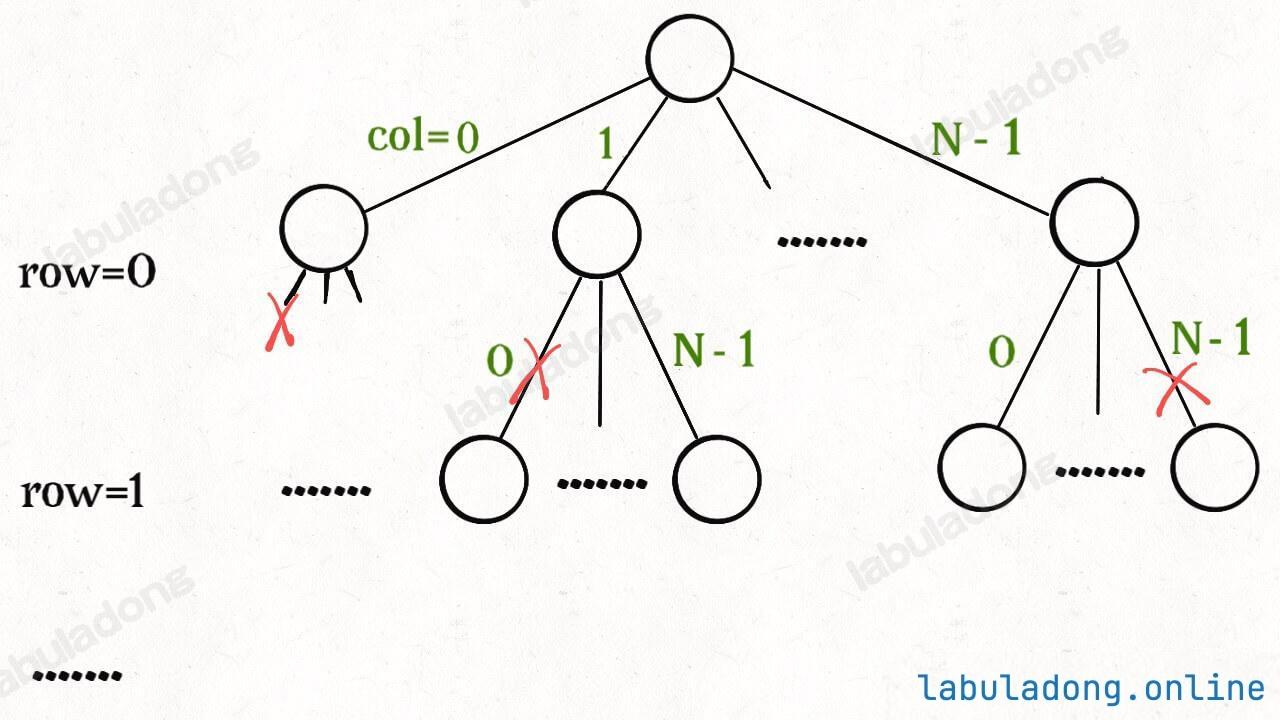`N = 8` 时，就是八皇后问题，数学大佬高斯穷尽一生都没有数清楚八皇后问题到底有几种可能的放置方法，但是我们的算法只需要一秒就可以算出来所有可能的结果。

`N` 行棋盘中，第一行有 `N` 个位置可能可以放皇后，第二行有 `N - 1` 个位置，第三行有 `N - 2` 个位置，以此类推，再叠加每次放皇后之前 `isValid` 函数所需的 O(N) 复杂度，所以总的时间复杂度上界是 O(N! * N)，而且没有什么明显的冗余计算可以优化效率。你可以试试 `N = 10` 的时候，计算就已经很耗时了。

``````bool found = false;
// 函数找到一个答案后就返回 true
bool backtrack(vector<string>& board, int row) {
if (found) {
// 已经找到一个答案了，不用再找了
return;
}

// 触发结束条件
if (row == board.size()) {
res.push_back(board);
// 找到了第一个答案
found = true;
return;
}

...
}
````````````// 仅仅记录合法结果的数量
int res = 0;

void backtrack(vector<string>& board, int row) {
if (row == board.size()) {
// 找到一个合法结果
res++;
return;
}

// 其他都一样
}
``````

### # 三、最后总结

``````def backtrack(...):
for 选择 in 选择列表:
做选择
backtrack(...)
撤销选择
``````

`backtrack` 函数时，需要维护走过的「路径」和当前可以做的「选择列表」，当触发「结束条件」时，将「路径」记入结果集